Over a million developers have joined DZone.{{announcement.body}}
{{announcement.title}}

# Bayesian Learning for Statistical Classification (Part 2)

DZone 's Guide to

# Bayesian Learning for Statistical Classification (Part 2)

### Learn just a few of the ways to use Bayes' theorem tools to help gain a foothold in the complex world of computational learning algorithms.

· AI Zone ·
Free Resource

Comment (0)

Save
{{ articles.views | formatCount}} Views

In Part 1, we got several examples of the use of Bayes’ theorem and probability in statistic classification. Let's conclude this 2-part series by talking about calibration, validation, and multi-class classification!

## Calibration

The nice thing about using a continuous decision function for binary classification is that it allows some degree of calibration. Consider the following classifier: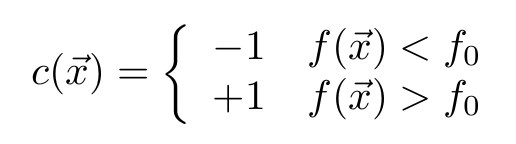Varying the classification threshold, f₀, allows us to adjust the sensitivity of the classifier. This is particularly useful in medical diagnostics.

Note that the case f=f₀ is left undefined. To remove bias, a random value should be returned when this occurs in numerical computations.

Suppose the classifier is trained using data with a prior class distribution of P’(i), while the population distribution is actually P(i). Assuming that f accurately estimates R, we want to find the value for f₀ such that the sample statistics are corrected to those of the population: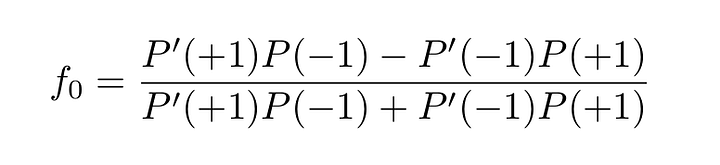Equation 12

To make this more detailed, consider the confusion matrix. The element of the confusion matrix in the ith row and jth column tells us, for all of the test data, in how many test samples had the ith class but the classifier returned the jth class.

By dividing by the number of test samples, the confusion matrix can be expressed as an approximate joint probability. Consider the confusion matrix for a binary classifier: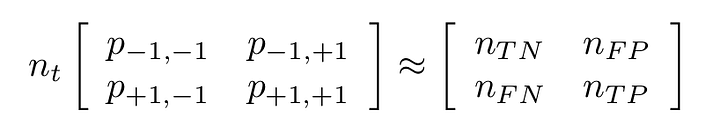• nt=nTN+nFP+nFN+nTP is the total number of test samples
• nTN is the number of true negatives
• nFP is the number of false positives
• nFN is the number of false negatives
• nTP is the number of true positives

A perfect classifier would return a diagonal matrix; an element is non-zero only when i=j.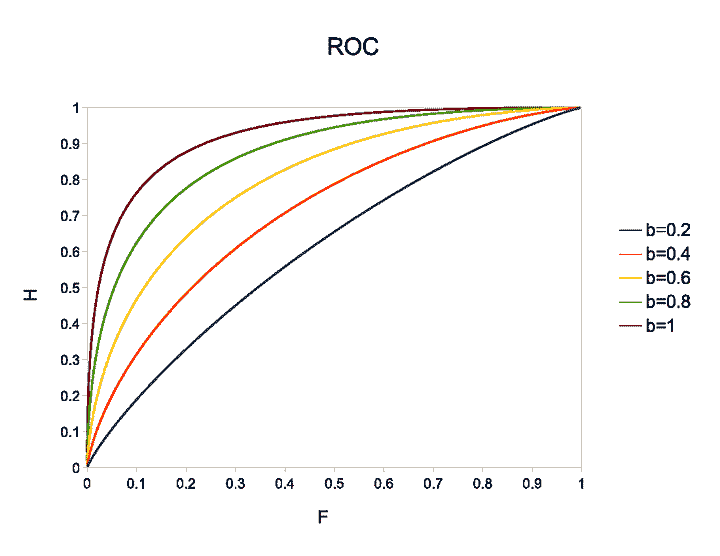From these five parameters, you can write down all possible skill scores for a simple binary classifier. The receiver operating characteristic (ROC) curve is produced by plotting two such skill scores against one another while varying the classification threshold. These are the hit rate: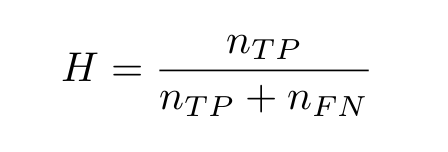And the false alarm rate: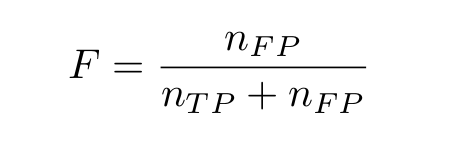The figure plots the ROC curve for the one-dimensional logistic classifier in (9) for h=1 and for different values of b. The classifier is assumed to be a perfect estimator for the conditional probabilities.

A more sophisticated calibration exercise would transform the decision function such that it accurately represents the difference in conditional probabilities. Consider the following equation, derived strictly from the background material presented in the first two sections: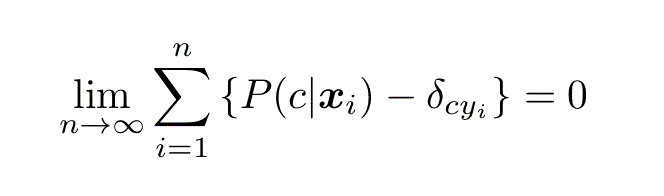Equation 13

Where δ is the Dirac delta function: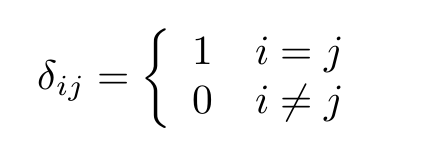A well-calibrated estimator for the conditional probabilities should obey this equation.

## Validation

Once we have derived a statistical classifier, we need to validate it on some test data. This data should be different from that used to train the classifier; otherwise, skill scores will be unduly optimistic. This is known as cross-validation.

The confusion matrix expresses everything about the accuracy of a discrete classifier over a given database and you can use it to compose any possible skill score. Here, we are going to cover two that are rarely seen in the literature but that are nonetheless important for reasons that will become clear.

The most basic skill score is accuracy: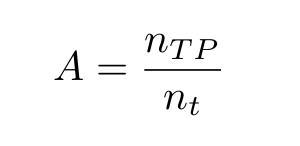With a maximum-likelihood classification algorithm, accuracy will be maximized. Accuracy, however, has several limitations that can be mitigated by using the following alternative measures.

The first is the uncertainty coefficient. This measure is based on Shannon’s channel capacity and requires, first, a definition of the information entropy. For a discrete probability, this is: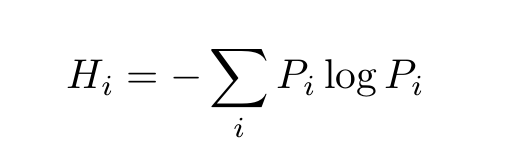This tells us how many bits we need to represent given that its prior distribution is Pᵢ. The measure can be extended to multivariate distributions. The conditional entropy is given: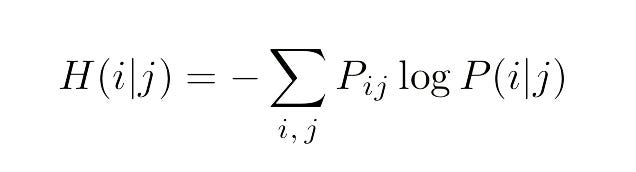Once we have these two definitions out of the way, we can write down the uncertainty coefficient: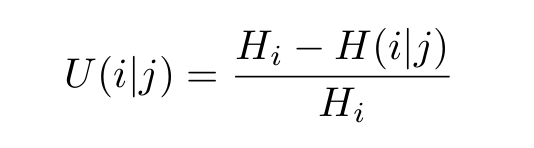Equation 14

which tells us how many bits of information a single classification result in j gives us of the true class value, i. This makes it a good skill score since the lowest possible value is 0, meaning the classifier provides, on average, no information on the true class values, while the highest is 1, meaning the classifier provides full information.

For binary classifiers, I also recommend the Pearson correlation coefficient: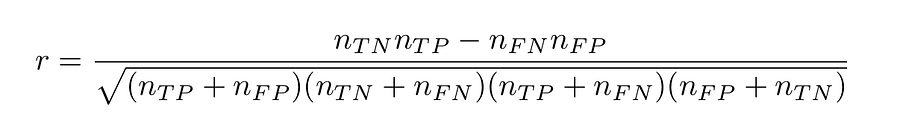Equation 15

Finally, for binary classifiers that return a continuum decision function rather than a discrete, binary value, we can use the ROC curve to measure the average skill for all possible thresholds by calculating the area under the curve.

For a perfect discriminator, the ROC curve will follow the unit square, rising to H=1 at F=0 and staying there for the duration, thus the area will be 1. An area of 0 is also a perfect classifier, but the sign is reversed, while a classifier with no discrimination value will follow the diagonal with an area of 0.5.

Note, for instance, how the area under the example curves gets larger as the separation between the classes increases.

## Multi-Class Classification

We have spent a considerable amount of time discussing binary classifiers. Assuming the only suitable statistical classifier we have at our disposal is a binary classifier, how do we generalize it to classification problems with more than two classes, that is, multi-class classifiers? We can use probability theory to derive an answer.

Suppose we design a set of binary classifiers by multiply partitioning the classes into two sets. A coding matrix A describes how this partitioning is done — the ith row of the matrix describes the partitioning of the ith binary classifier with a -1/+1 in the jth column, meaning that the jth class label was transformed to a -1/+1 for the training and a 0, meaning it was excluded entirely.

The conditional probabilities of the multi-class problem are related to those of the binary classifiers as follows: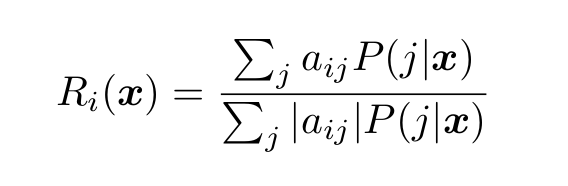Equation 16

With some rearrangement, we can transform this into a linear system: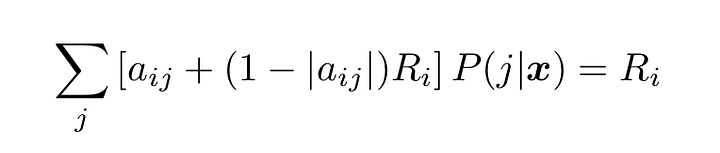Equation 17

...where Ri is the difference in conditional probabilities for the ith binary classifier.

As an example, consider the “one-versus-the-rest” approach to multi-class classification. Here, we compare each class with all the others. The coding matrix is given (similar to the Dirac delta function):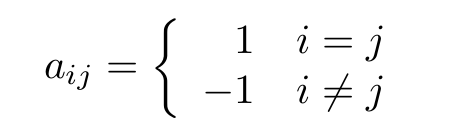Equation 18

The preceding assumes that the conditional probabilities for the binary classifiers are estimated correctly. Otherwise, we need to constrain the resulting multi-class probabilities. Neglecting the second argument, a conditional probability has the same properties as a univariate probability. First, they all ought to sum to one: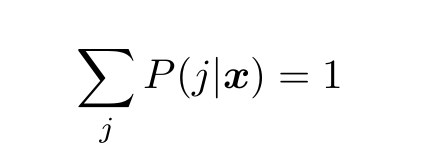Equation 19

Second, they should all be positive: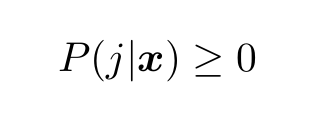The normalization constraint in Equation 18, being an equality constraint, is the easiest to enforce.

One way is to introduce a “slack” variable: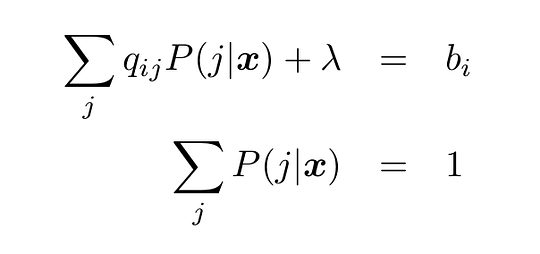Equation 20

...where Qp = b is the linear system for the unconstrained problem and �� is the slack variable.

For the “one-versus-one’’ method of multi-class classification, where we compare each class with each of the others in turn, this is all we need. It turns out that once the normalization constraint is enforced, all the others fall into place and the solution has only positive elements.

Note that because the system of equations is overdetermined, it will need to be solved as a least-squares problem and there is one other caveat: the normalization must be done separately from the least squares minimization.

In other words, we form the normal equations from Equation 17 first, then plug these into Equation 20. To learn about the normal equations, please see my upcoming article, “Mastering Singular Value Decomposition.”

## Problems

This list of problems is provided to help you with Bayesian learning and probability theory and derive useful formulas related to statistical classification. They will also get you thinking about some of the fundamental issues in the field.

1. Why does the fitting for the logistic classifier in Equation 10 have to be nonlinear? What advantage does this have?
2. Do some research online to find nonlinear optimization algorithms to fit the logistic classifier.
3. Derive Equation 12. (It’s surprisingly difficult.) How important do you think it is, on average, to correct for the class distribution? Explain.
4. How would you calculate the ROC curves shown in the figure? Fill in the missing steps going from Equation 8 to Equation 9 and then to the calculation of the ROC curves.
5. Derive Equation 13.
6. List the advantages of the uncertainty coefficient and the correlation coefficient (for binary classifiers) as a measure of classification skill. What happens when:
• The class labels are rearranged?
• The distribution of the class labels is changed in the test data? How does this affect the outcome?
7. From the general formula for Pearson correlation, derive Equation 15. Note: This is not trivial.
8. Correlation is normally not appropriate for multi-class classification problems. Why not? What types of problems would be the exceptions?
9. Derive Equation 17 from Equation 16. Hint: What property of P(i|x̄) do you need to complete the derivation?
10. The one-versus-the-rest coding matrix in Equation 18 can use a simplified version of Equation 17. Explain.
11. Write down the coding matrix for the one-versus-one multi-class classifier.
12. Find some statistical classification data online or create some on your own; for example, by classifying pixels in an image. Perform statistical classifications by fitting a multi-dimensional Gaussian to each of the classes: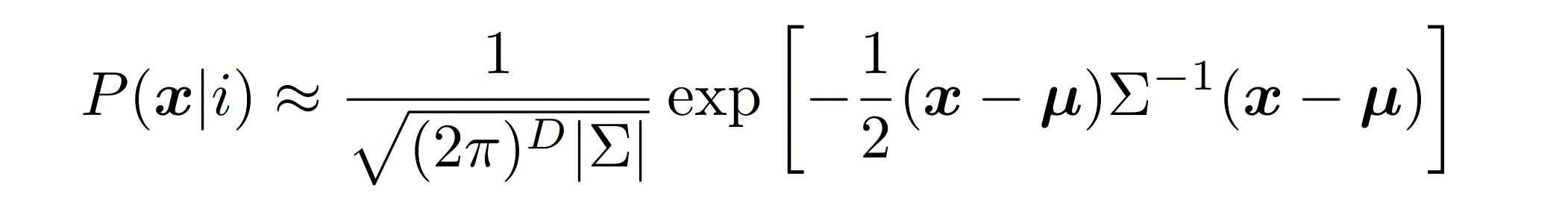Where Σ is the covariance matrix, ��  is the arithmetic mean, and D is the dimension of the features data. Measure the accuracy of your results. Don’t forget to divide the data into a test set and a training set.

## Conclusion

I hope you got the idea of Bayesian learning for statistical classification. Mathematical models are not closed systems. They can be expanded, re-purposed, and recombined.

The applications of probability and Bayes’ theorem and the problems we can put them to are limited only by the imagination. Here, we have presented just a few of the ways to use these tools to help gain a foothold in the complex world of computational learning algorithms.

Topics:
ai ,algorithms ,machine learning ,statistics ,tutorial ,bayes theorem

Comment (0)

Save
{{ articles.views | formatCount}} Views

Published at DZone with permission of

Opinions expressed by DZone contributors are their own.